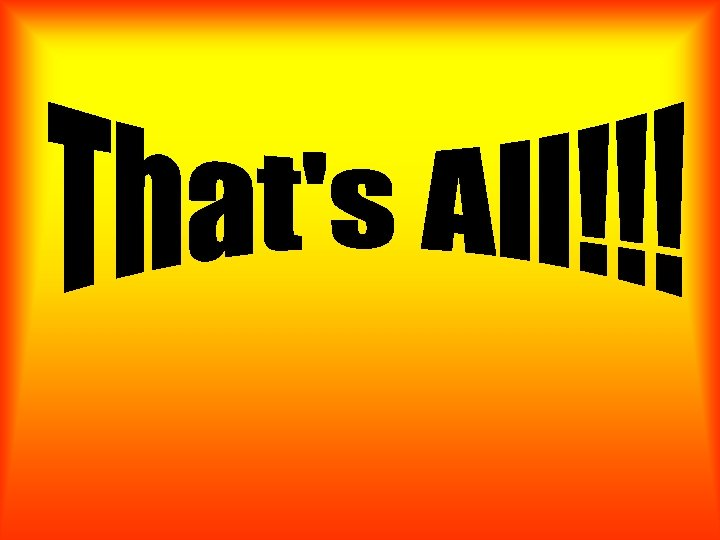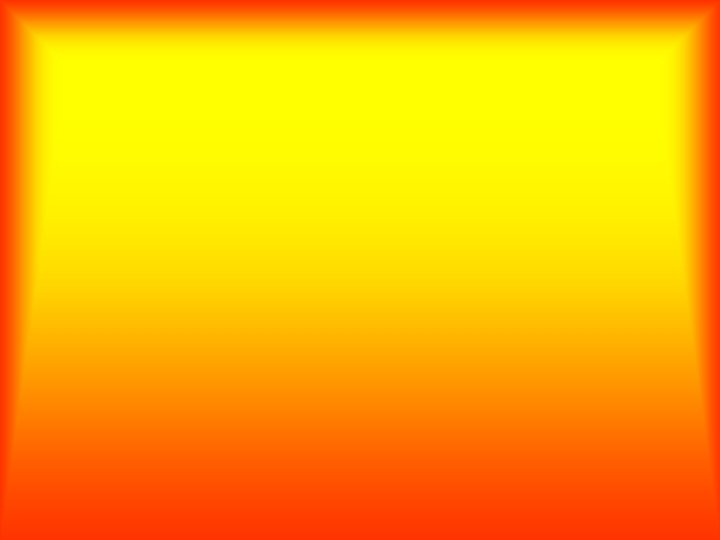Скачать презентацию Whole Class Review Activity Volume and Capacity

42009f88281dfbce37c82cb119f96941.ppt

• Количество слайдов: 52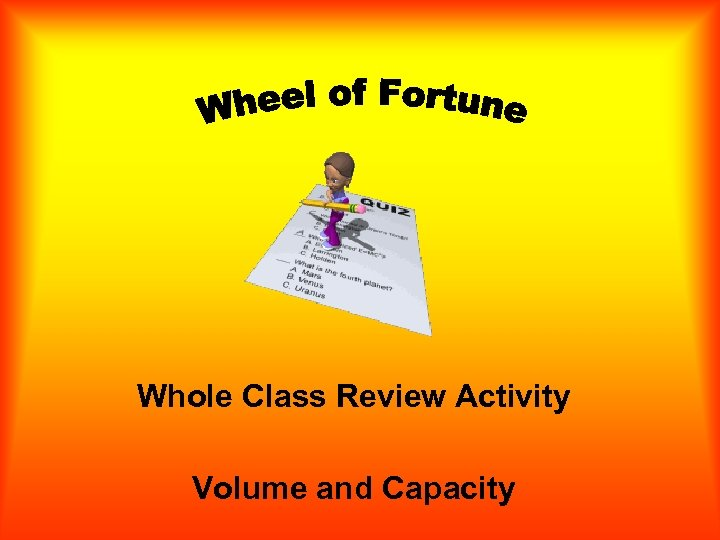Whole Class Review Activity Volume and Capacity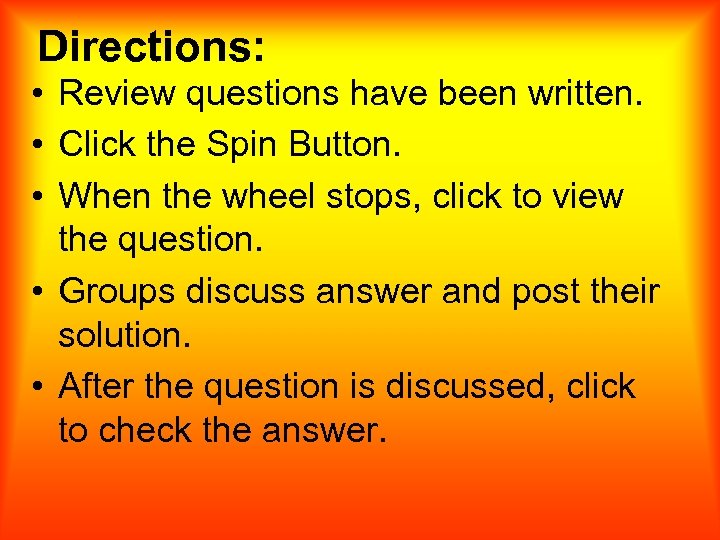Directions: • Review questions have been written. • Click the Spin Button. • When the wheel stops, click to view the question. • Groups discuss answer and post their solution. • After the question is discussed, click to check the answer.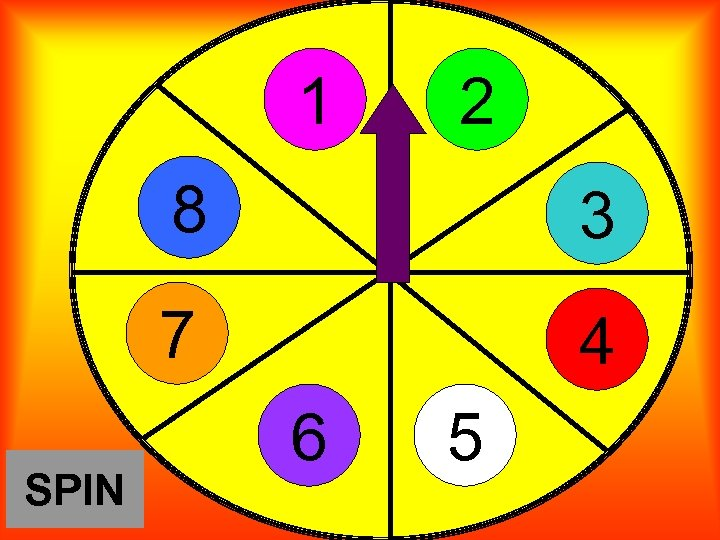1 2 8 7 SPIN 3 4 6 5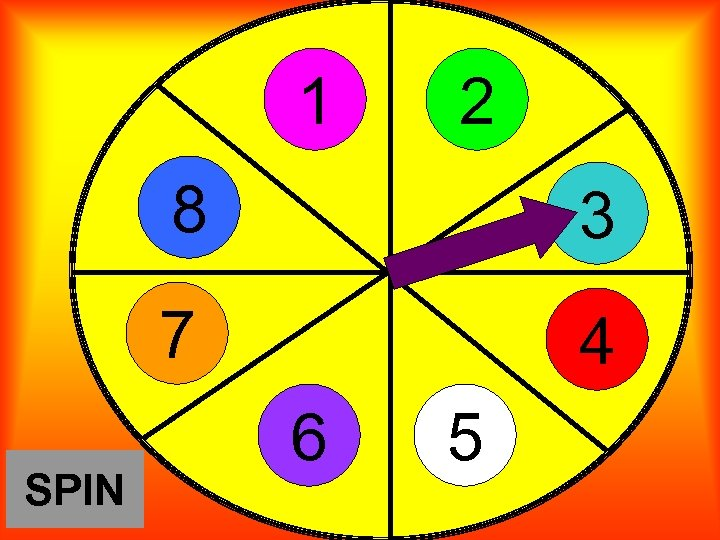1 2 8 7 SPIN 3 4 6 5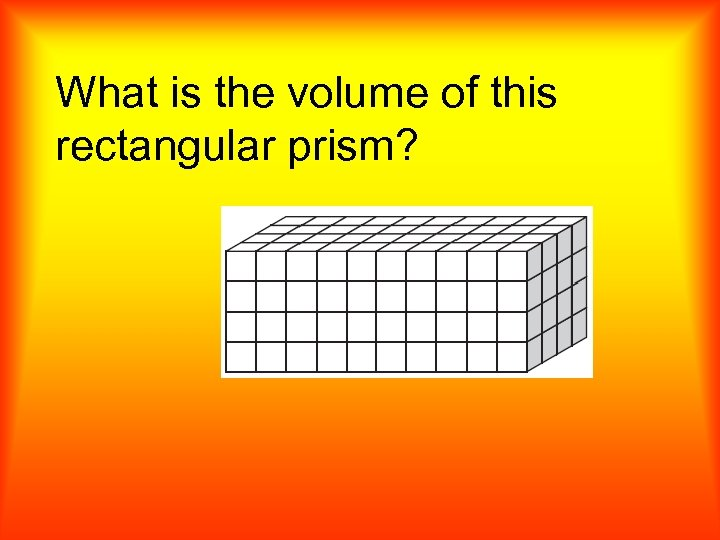What is the volume of this rectangular prism?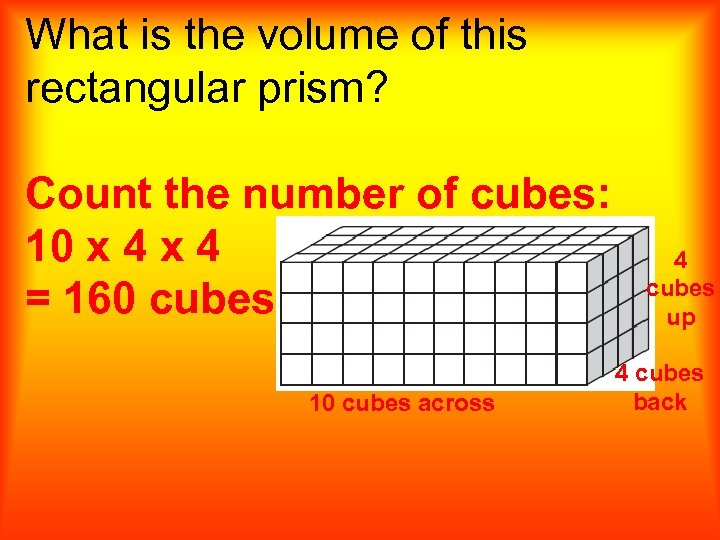What is the volume of this rectangular prism? Count the number of cubes: 10 x 4 = 160 cubes 10 cubes across 4 cubes up 4 cubes back1 2 8 7 SPIN 3 4 6 5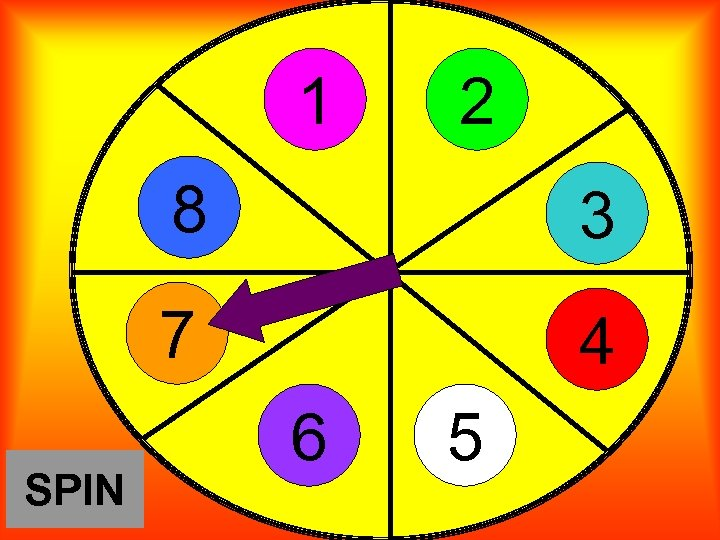1 2 8 7 SPIN 3 4 6 5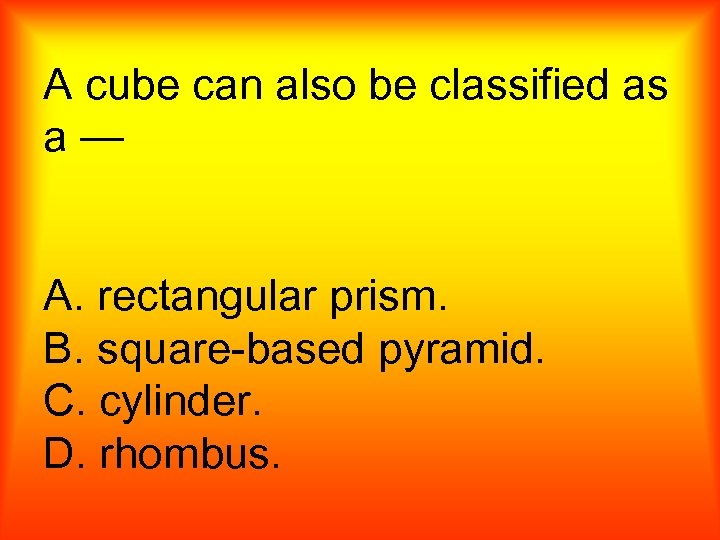A cube can also be classified as a— A. rectangular prism. B. square-based pyramid. C. cylinder. D. rhombus.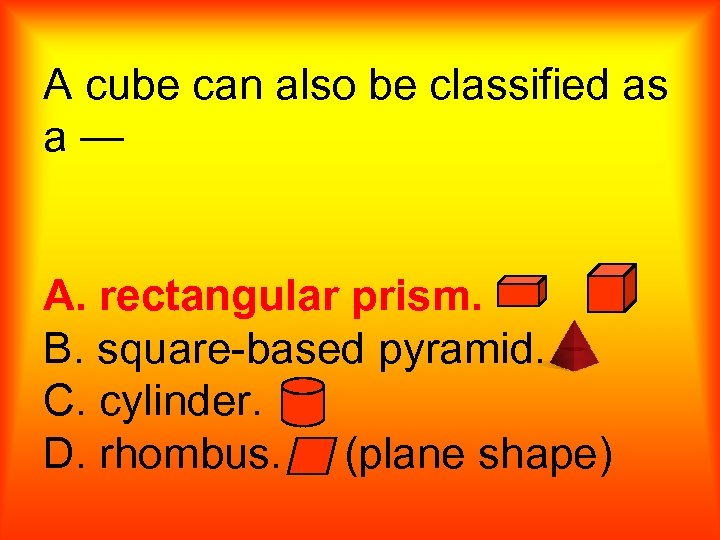A cube can also be classified as a— A. rectangular prism. B. square-based pyramid. C. cylinder. D. rhombus. (plane shape)1 2 8 7 SPIN 3 4 6 5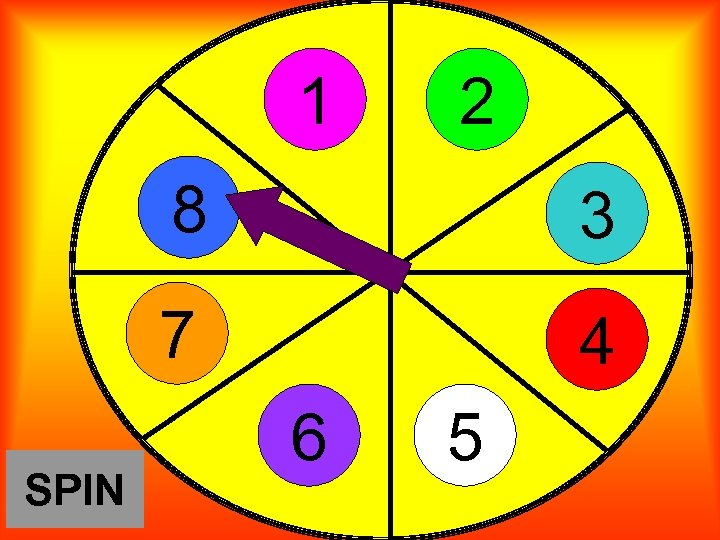1 2 8 7 SPIN 3 4 6 5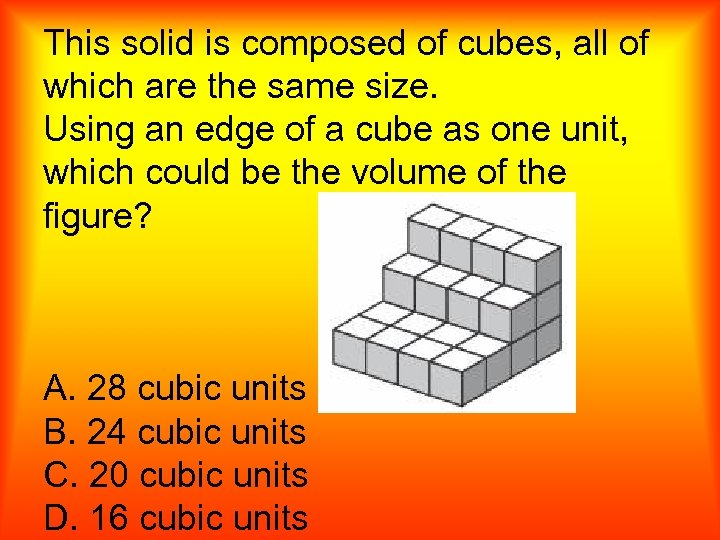This solid is composed of cubes, all of which are the same size. Using an edge of a cube as one unit, which could be the volume of the figure? A. 28 cubic units B. 24 cubic units C. 20 cubic units D. 16 cubic units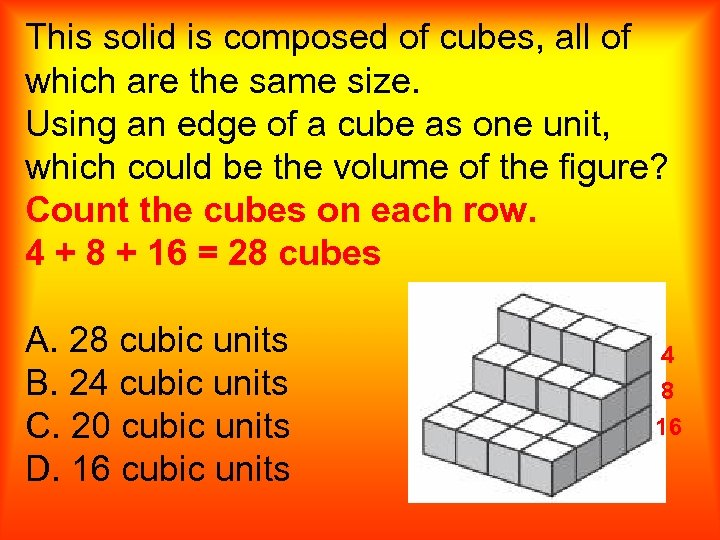This solid is composed of cubes, all of which are the same size. Using an edge of a cube as one unit, which could be the volume of the figure? Count the cubes on each row. 4 + 8 + 16 = 28 cubes A. 28 cubic units B. 24 cubic units C. 20 cubic units D. 16 cubic units 4 8 161 2 8 7 SPIN 3 4 6 5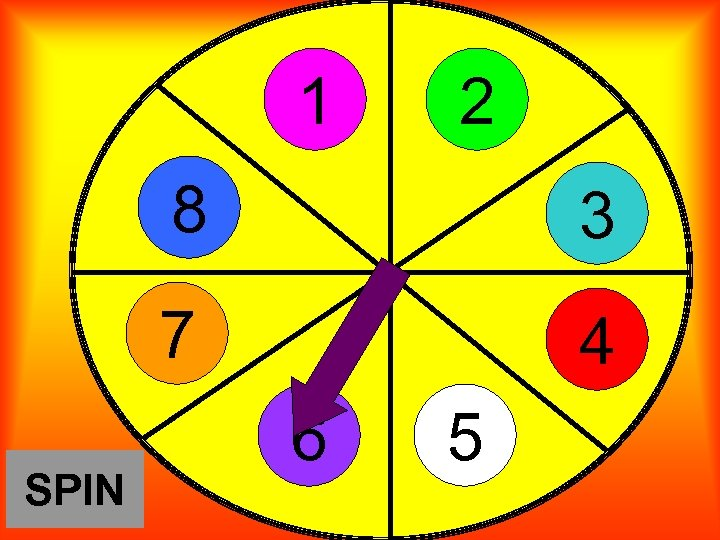1 2 8 7 SPIN 3 4 6 5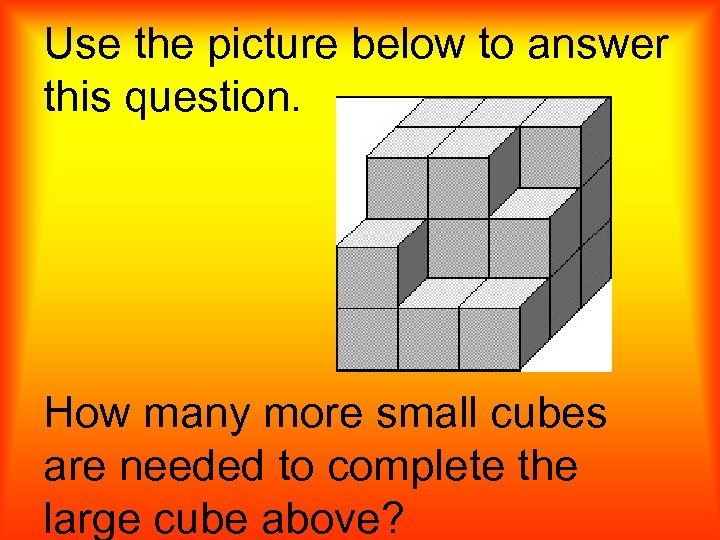Use the picture below to answer this question. How many more small cubes are needed to complete the large cube above?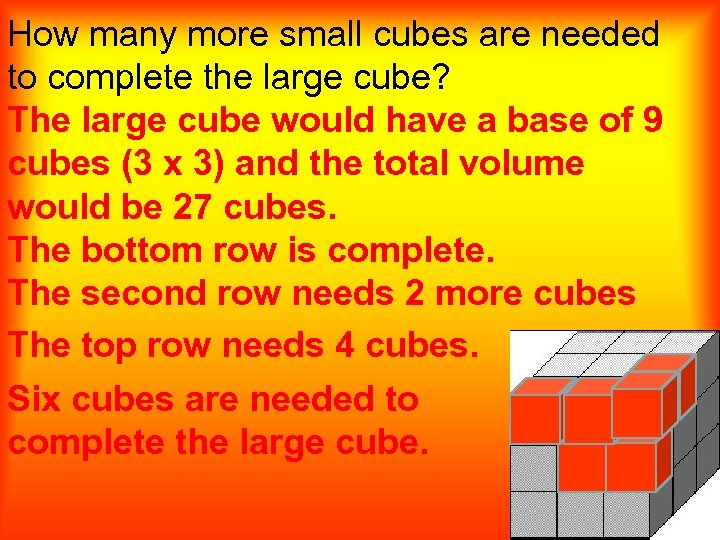How many more small cubes are needed to complete the large cube? The large cube would have a base of 9 cubes (3 x 3) and the total volume would be 27 cubes. The bottom row is complete. The second row needs 2 more cubes The top row needs 4 cubes. Six cubes are needed to complete the large cube.1 2 8 7 SPIN 3 4 6 5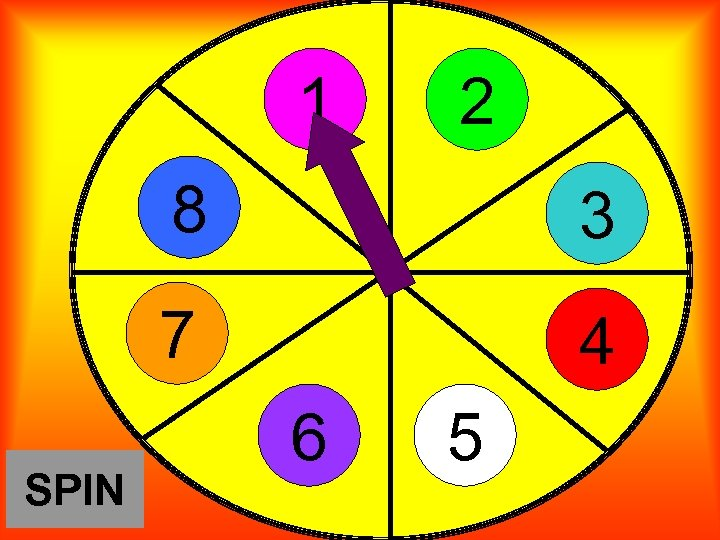1 2 8 7 SPIN 3 4 6 5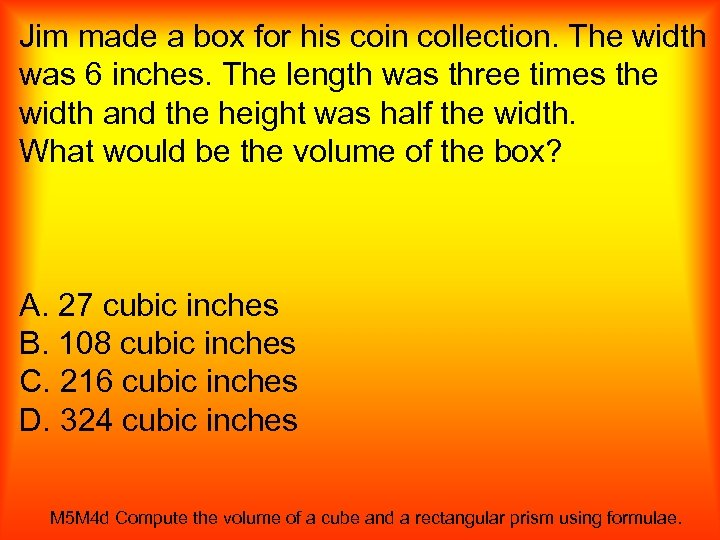Jim made a box for his coin collection. The width was 6 inches. The length was three times the width and the height was half the width. What would be the volume of the box? A. 27 cubic inches B. 108 cubic inches C. 216 cubic inches D. 324 cubic inches M 5 M 4 d Compute the volume of a cube and a rectangular prism using formulae.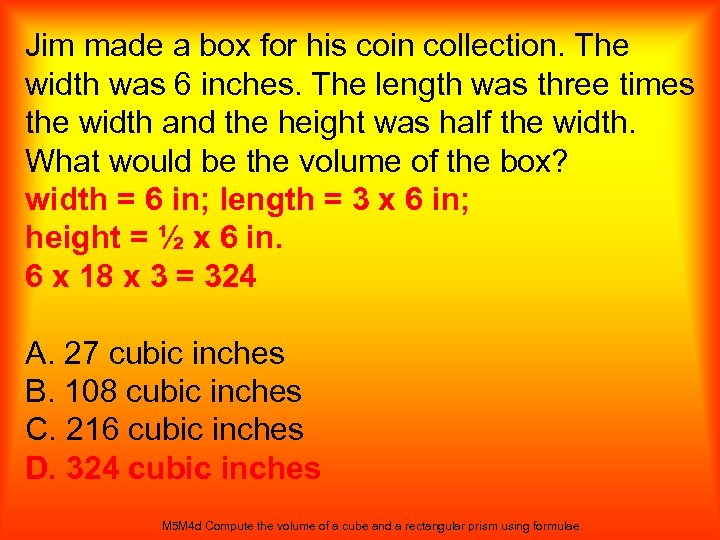Jim made a box for his coin collection. The width was 6 inches. The length was three times the width and the height was half the width. What would be the volume of the box? width = 6 in; length = 3 x 6 in; height = ½ x 6 in. 6 x 18 x 3 = 324 A. 27 cubic inches B. 108 cubic inches C. 216 cubic inches D. 324 cubic inches M 5 M 4 d Compute the volume of a cube and a rectangular prism using formulae.1 2 8 7 SPIN 3 4 6 5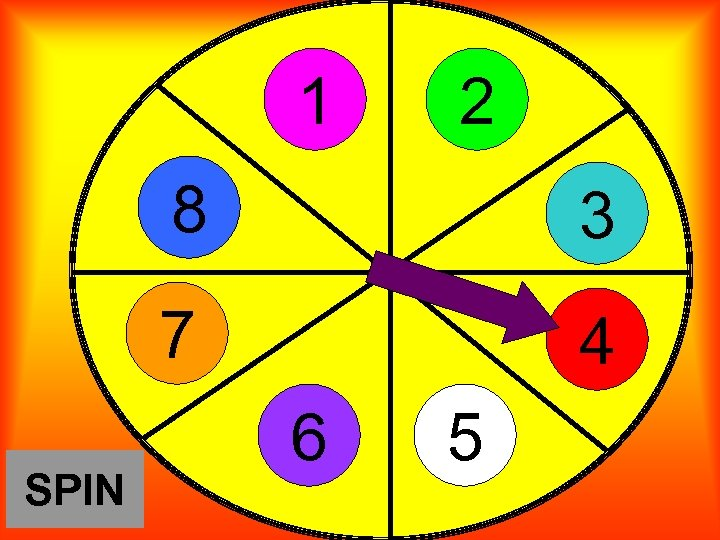1 2 8 7 SPIN 3 4 6 5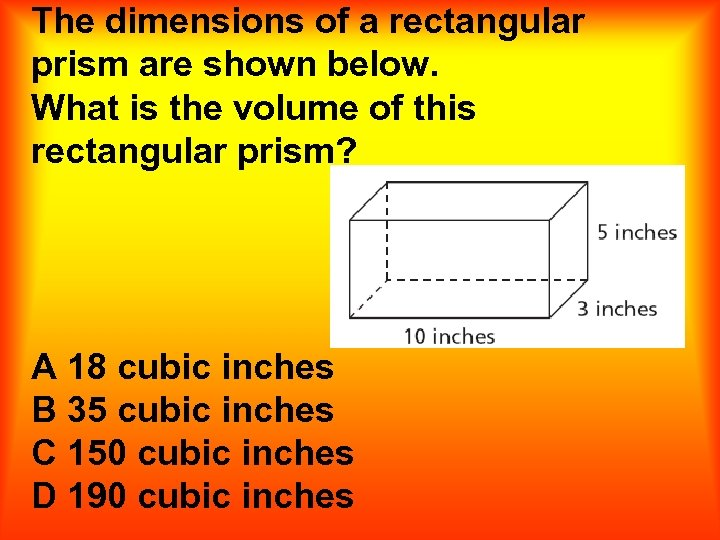The dimensions of a rectangular prism are shown below. What is the volume of this rectangular prism? A 18 cubic inches B 35 cubic inches C 150 cubic inches D 190 cubic inches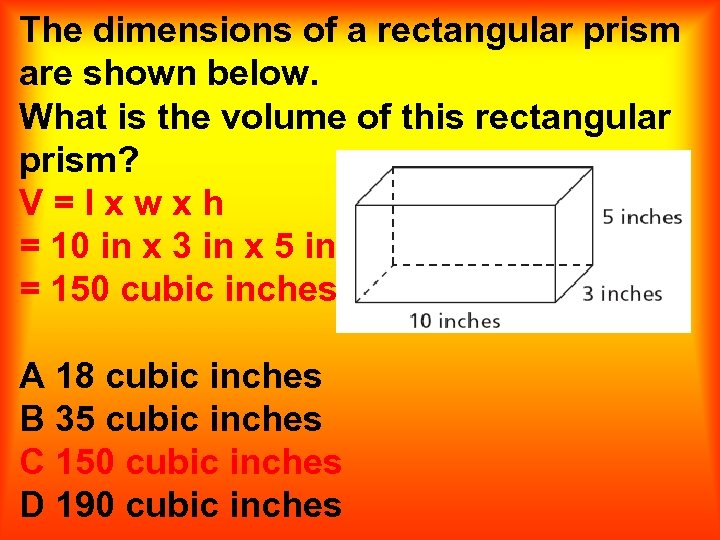The dimensions of a rectangular prism are shown below. What is the volume of this rectangular prism? V=lxwxh = 10 in x 3 in x 5 in = 150 cubic inches A 18 cubic inches B 35 cubic inches C 150 cubic inches D 190 cubic inches1 2 8 7 SPIN 3 4 6 5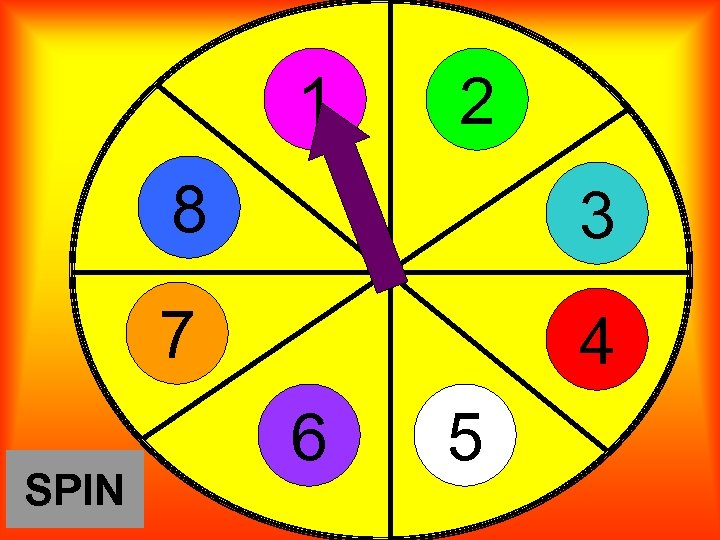1 2 8 7 SPIN 3 4 6 5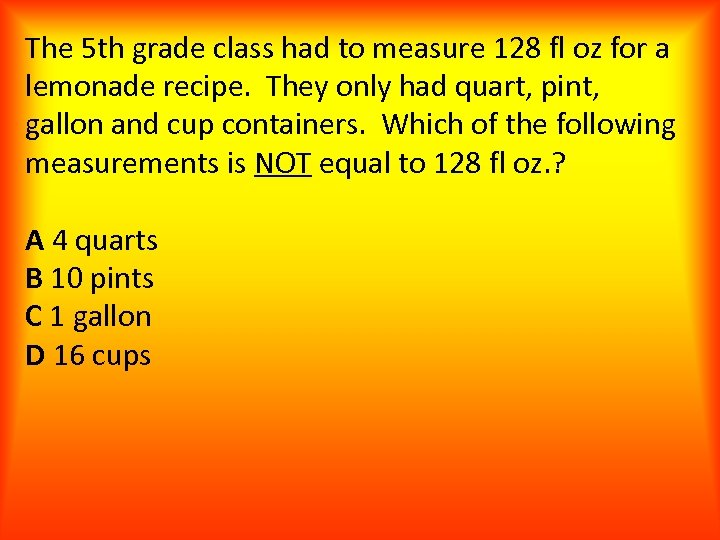The 5 th grade class had to measure 128 fl oz for a lemonade recipe. They only had quart, pint, gallon and cup containers. Which of the following measurements is NOT equal to 128 fl oz. ? A 4 quarts B 10 pints C 1 gallon D 16 cups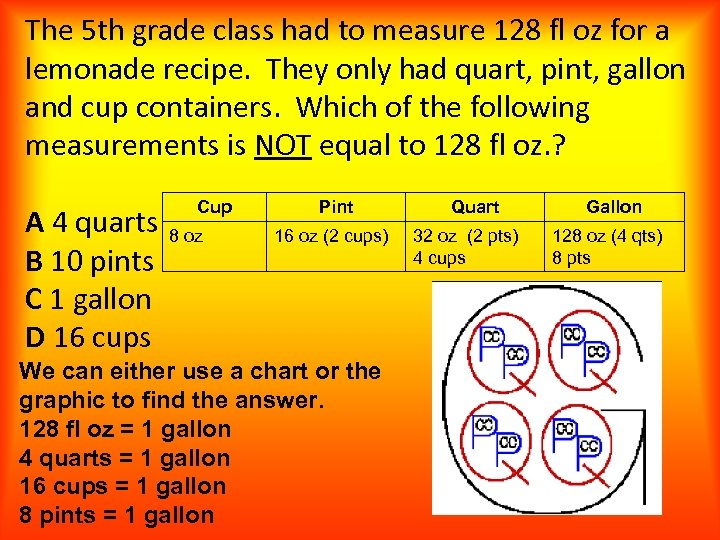The 5 th grade class had to measure 128 fl oz for a lemonade recipe. They only had quart, pint, gallon and cup containers. Which of the following measurements is NOT equal to 128 fl oz. ? A 4 quarts B 10 pints C 1 gallon D 16 cups Cup 8 oz Pint 16 oz (2 cups) We can either use a chart or the graphic to find the answer. 128 fl oz = 1 gallon 4 quarts = 1 gallon 16 cups = 1 gallon 8 pints = 1 gallon Quart 32 oz (2 pts) 4 cups Gallon 128 oz (4 qts) 8 pts1 2 8 7 SPIN 3 4 6 5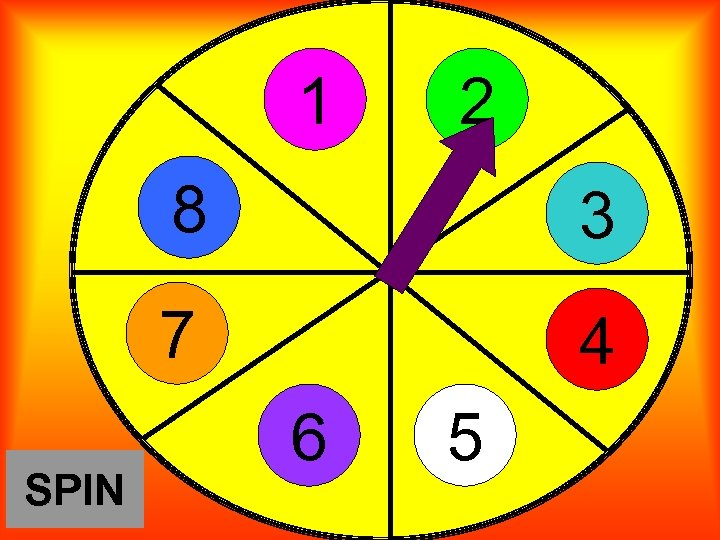1 2 8 7 SPIN 3 4 6 5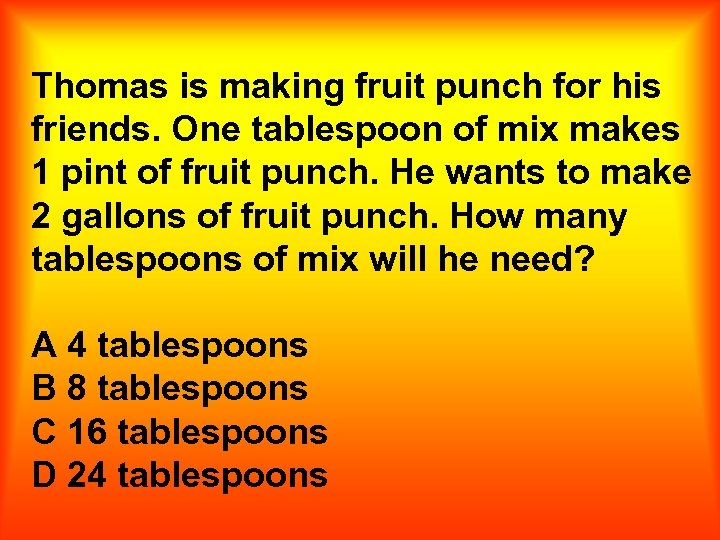Thomas is making fruit punch for his friends. One tablespoon of mix makes 1 pint of fruit punch. He wants to make 2 gallons of fruit punch. How many tablespoons of mix will he need? A 4 tablespoons B 8 tablespoons C 16 tablespoons D 24 tablespoons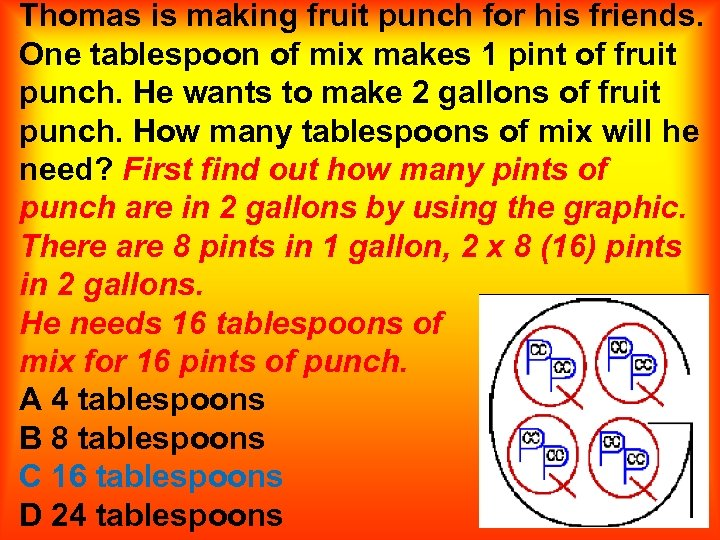Thomas is making fruit punch for his friends. One tablespoon of mix makes 1 pint of fruit punch. He wants to make 2 gallons of fruit punch. How many tablespoons of mix will he need? First find out how many pints of punch are in 2 gallons by using the graphic. There are 8 pints in 1 gallon, 2 x 8 (16) pints in 2 gallons. He needs 16 tablespoons of mix for 16 pints of punch. A 4 tablespoons B 8 tablespoons C 16 tablespoons D 24 tablespoons1 2 8 7 SPIN 3 4 6 5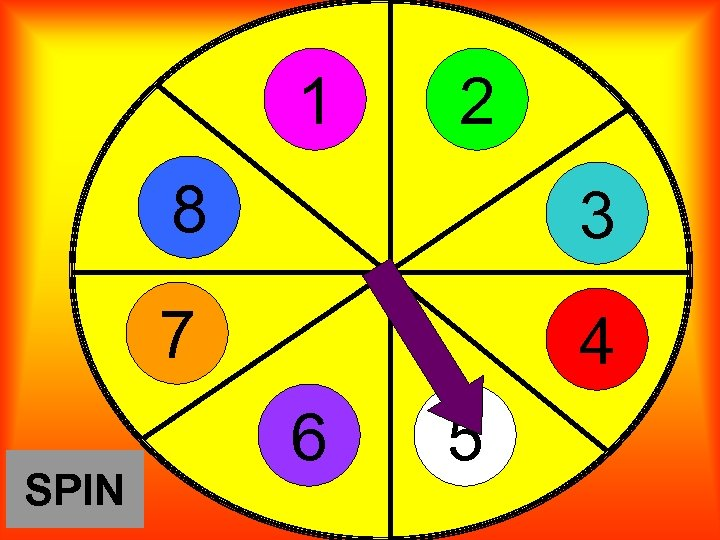1 2 8 7 SPIN 3 4 6 5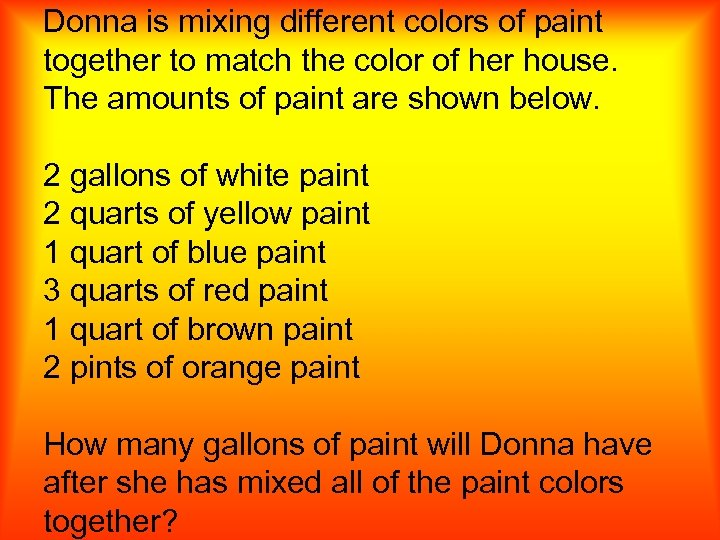Donna is mixing different colors of paint together to match the color of her house. The amounts of paint are shown below. 2 gallons of white paint 2 quarts of yellow paint 1 quart of blue paint 3 quarts of red paint 1 quart of brown paint 2 pints of orange paint How many gallons of paint will Donna have after she has mixed all of the paint colors together?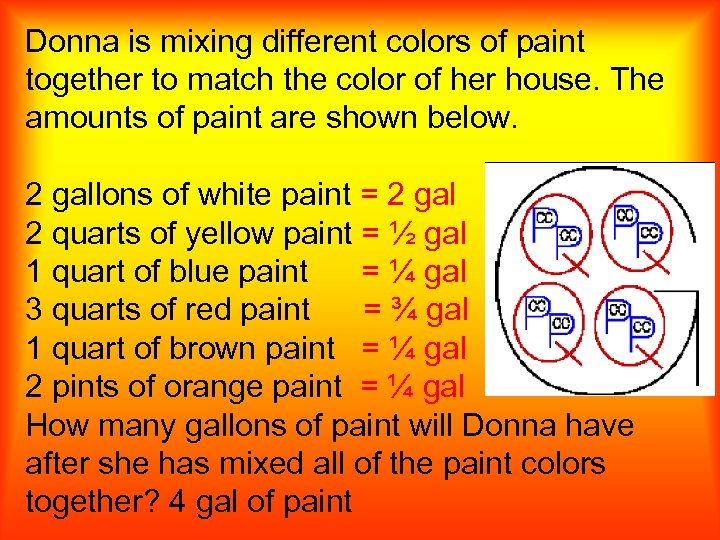Donna is mixing different colors of paint together to match the color of her house. The amounts of paint are shown below. 2 gallons of white paint = 2 gal 2 quarts of yellow paint = ½ gal 1 quart of blue paint = ¼ gal 3 quarts of red paint = ¾ gal 1 quart of brown paint = ¼ gal 2 pints of orange paint = ¼ gal How many gallons of paint will Donna have after she has mixed all of the paint colors together? 4 gal of paint1 2 8 7 SPIN 3 4 6 51 2 8 7 SPIN 3 4 6 5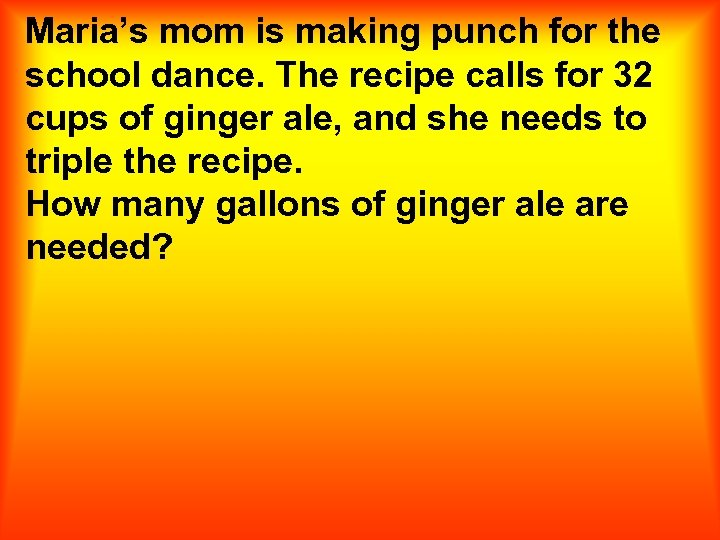Maria’s mom is making punch for the school dance. The recipe calls for 32 cups of ginger ale, and she needs to triple the recipe. How many gallons of ginger ale are needed?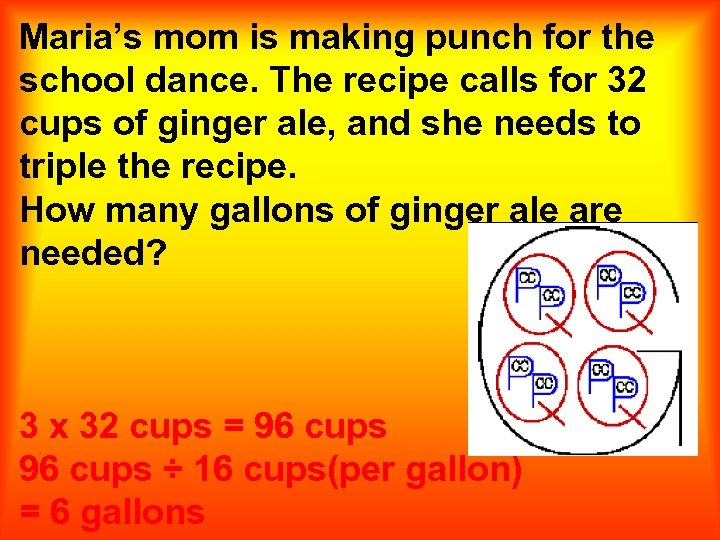Maria’s mom is making punch for the school dance. The recipe calls for 32 cups of ginger ale, and she needs to triple the recipe. How many gallons of ginger ale are needed? 3 x 32 cups = 96 cups ÷ 16 cups(per gallon) = 6 gallons1 2 8 7 SPIN 3 4 6 51 2 8 7 SPIN 3 4 6 5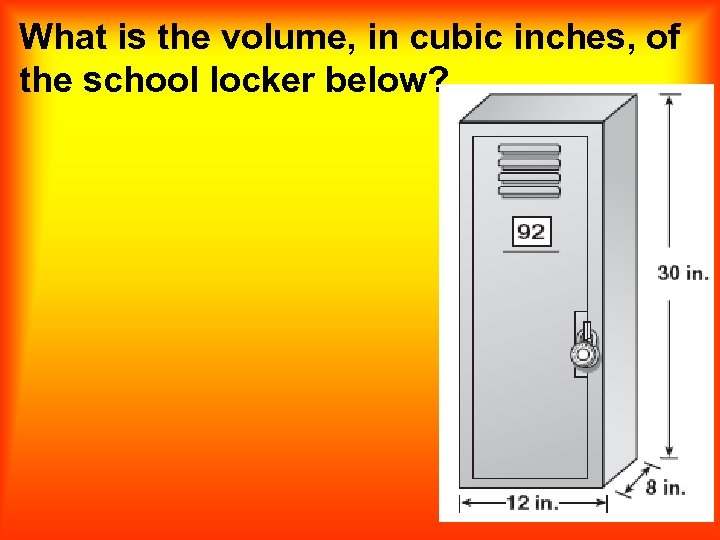What is the volume, in cubic inches, of the school locker below?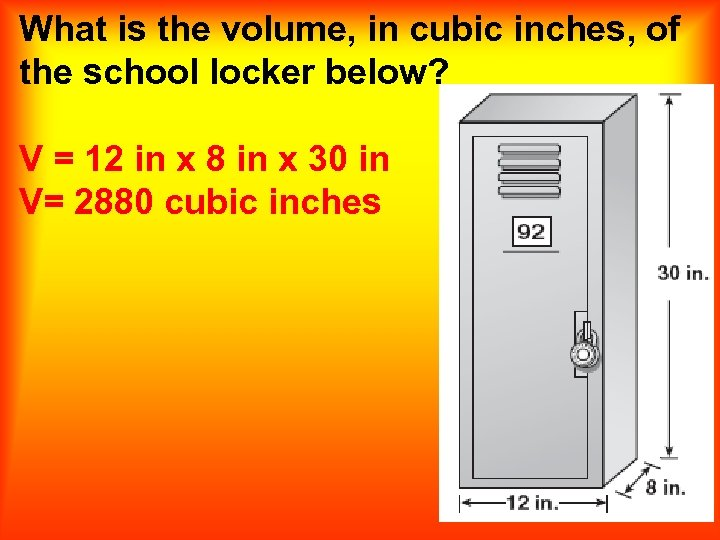What is the volume, in cubic inches, of the school locker below? V = 12 in x 8 in x 30 in V= 2880 cubic inches1 2 8 7 SPIN 3 4 6 51 2 8 7 SPIN 3 4 6 5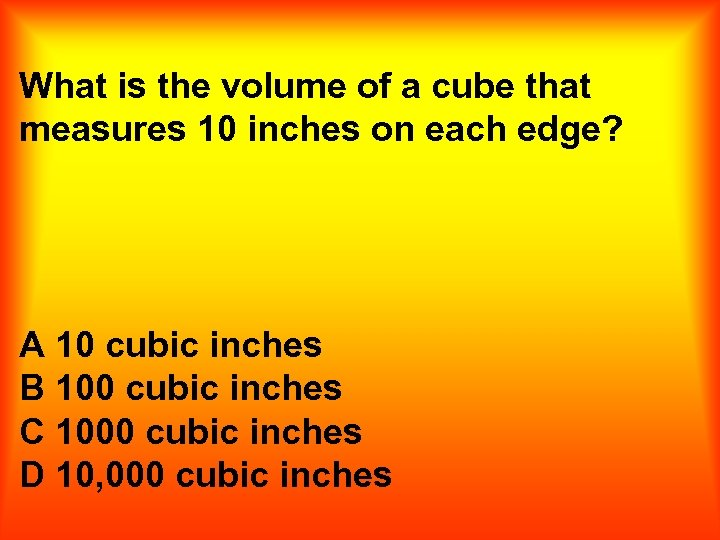What is the volume of a cube that measures 10 inches on each edge? A 10 cubic inches B 100 cubic inches C 1000 cubic inches D 10, 000 cubic inches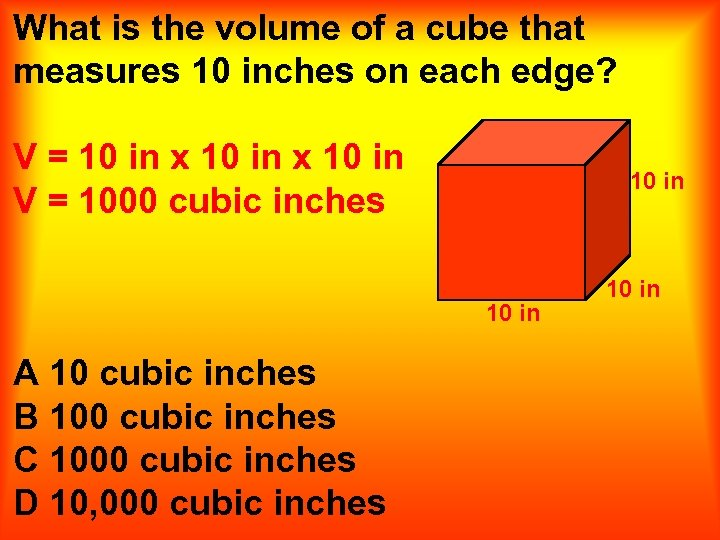What is the volume of a cube that measures 10 inches on each edge? V = 10 in x 10 in V = 1000 cubic inches 10 in A 10 cubic inches B 100 cubic inches C 1000 cubic inches D 10, 000 cubic inches 10 in